# Advanced Data Visualization for Data Science in Python

How to make cool, fully interactive graphics with one line of Python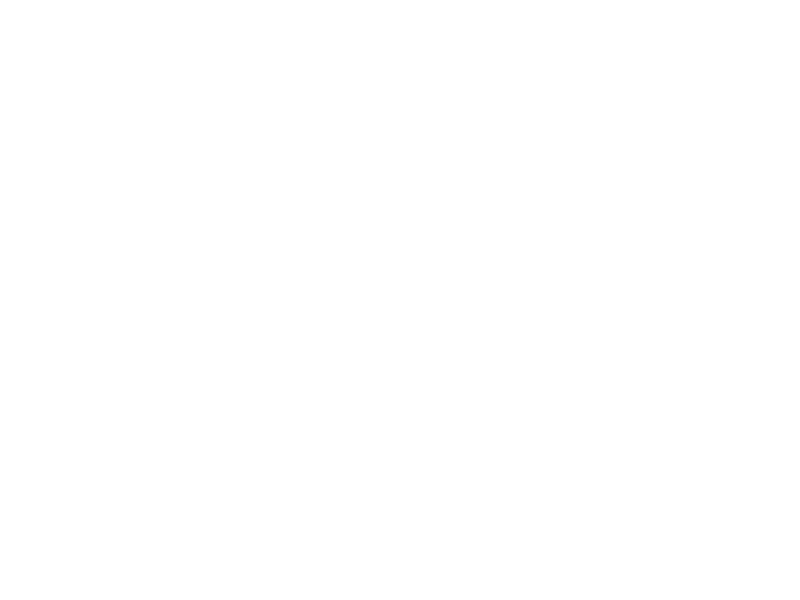Undervalued misconception is one of many harmful cognitive biasesvictims of which are people. It belongs to our tendency to continue to devote time and resources to the lost cause, because we have already spent – drowned – so much time in pursuit. The undervalued misconception applies to staying on a bad job longer than we should, slavishly working on a project, even when it is clear that it will not work, and yes, continuing to use the tedious, outdated charting library – matplotlib – when there are more effective, interactive, and more attractive alternatives.

Over the past few months, I realized that the only reason I use matplotlib is the hundreds of hours I spent on learning complex syntax. These difficulties lead to hours of frustration by figuring out on StackOverflow how format dates or add second y axis. Fortunately, this is a great time to plot in Python, and after exploring options, the clear winner – in terms of ease of use, documentation and functionality – is plotly library. In this article, we dive right into plotly, learning how to create better graphics in less time — often with a single line of code.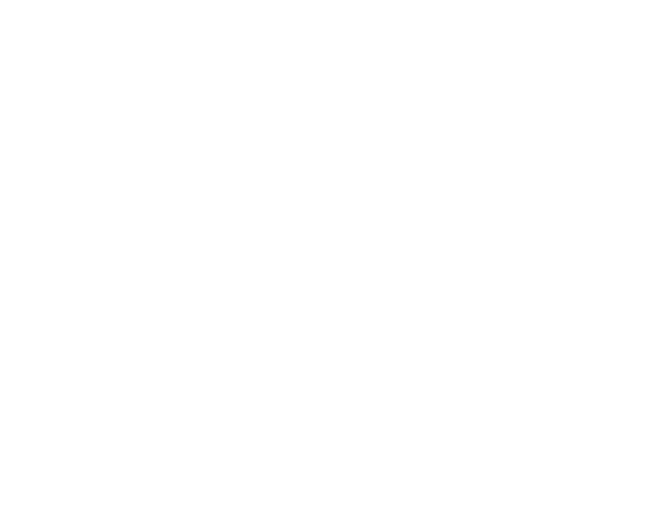### Plotly Overview

Package plotly for Python is an open source library, built on plotly.jswhich, in turn, is built on d3.js. We will use a wrapper over plotly called cufflinksdesigned to work with Pandas DataFrame So, our stack cufflinks> plotly> plotly.js> d3.js – this means that we get the efficiency of programming in Python with incredible interactive graphics capabilities d3.

(Itself Plotly – graphic company with multiple open source products and tools. The Python library is free to use, and we can create an unlimited number of diagrams offline plus up to 25 diagrams online to share them with the whole world.)

All of the work in this article was done on a Jupyter Notebook with plotly + cufflinks working offline. After installing plotly and cufflinks with `pip install cufflinks plotly `import the following to run in jupiter:

``````# Standard plotly imports
import plotly.plotly as py
import plotly.graph_objs as go
from plotly.offline import iplot, init_notebook_mode
# Using plotly + cufflinks in offline mode
init_notebook_mode(connected=True)``````

### Distributions of single variables: histograms and box rafts

Charts with one variable – one-dimensional – are the standard way to start the analysis, and the histogram is the transition graph (although with some problems) to plot the distribution. Here, using my average article statistics (you can see how get your own stats here or use mine), let’s make an interactive histogram of the number of pops on articles ( `df` Is a standard Pandas data frame):

``````df['claps'].iplot(kind='hist', xTitle="claps",
yTitle="count", title="Claps Distribution")``````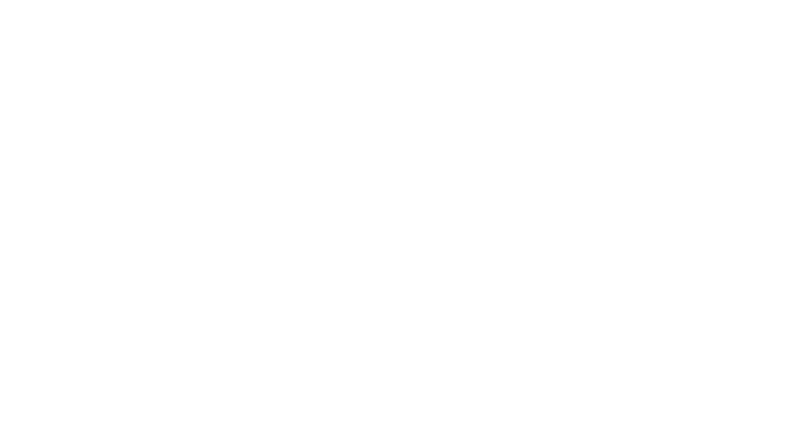For those who are used to `matplotlib`, all we need to do is add another letter (`iplot` instead `plot`), and we will get a much more beautiful and interactive chart! We can click on the data in order to receive more detailed information, increase the scale of the chart sections and, as we will see later, select different categories.

If we want to build superimposed histograms, it is just as simple:

``````df[['time_started', 'time_published']].iplot(
kind='hist',
histnorm='percent',
barmode="overlay",
xTitle="Time of Day",
yTitle="(%) of Articles",
title="Time Started and Time Published")``````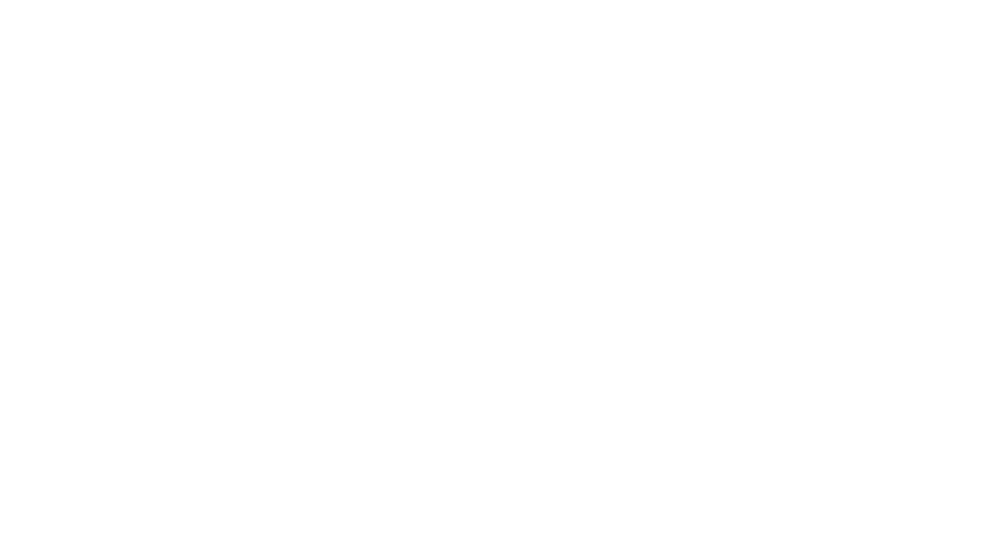A little manipulation `Pandas`we can make a barplot:

``````# Resample to monthly frequency and plot
set_index('published_date').
resample('M').mean()
df2.iplot(kind='bar', xTitle="Date", yTitle="Average",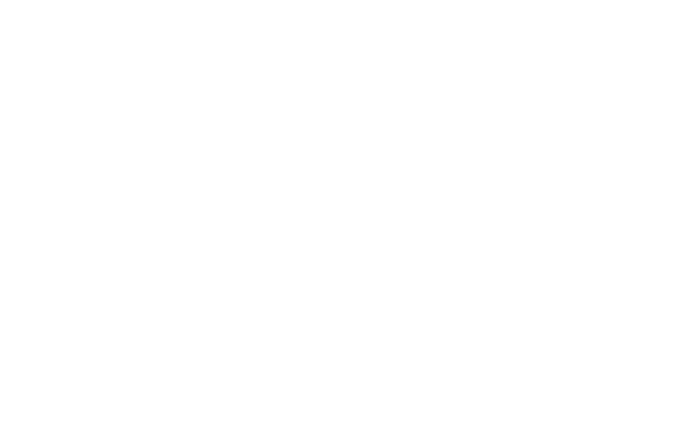as we have seen, we can combine force pandas with plotly + cufflinks. For boxplot distribution of fans by publication, we use `pivot`and then plot:

``````df.pivot(columns="publication", values="fans").iplot(
kind='box',
yTitle="fans",
title="Fans Distribution by Publication")``````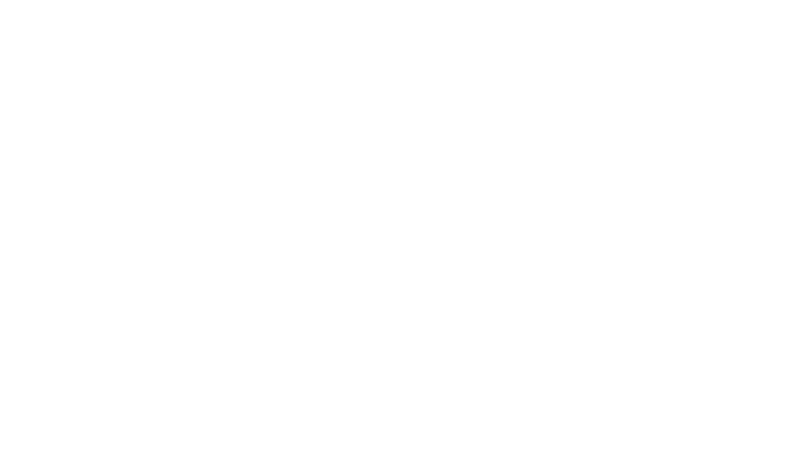The benefits of interactivity are that we can research and post data as we see fit. There is a lot of information in the box raft, and without the ability to see the numbers, we will miss most of it!

### Scatter chart

The scatter plot is the heart of most analyzes. This allows us to see the evolution of a variable over time or the relationship between two (or more) variables.

### Time series

A significant part of the real data has an element of time. Fortunately, plotly + cufflinks was designed with time series visualization in mind. Let’s make a data frame from my TDS articles and see how trends have changed.

`````` Create a dataframe of Towards Data Science Articles
tds = df[df['publication'] == 'Towards Data Science'].
set_index('published_date')
# Plot read time as a time series
tds[['claps', 'fans', 'title']].iplot(
y='claps', mode="lines+markers", secondary_y = 'fans',
secondary_y_title="Fans", xTitle="Date", yTitle="Claps",
text="title", title="Fans and Claps over Time")``````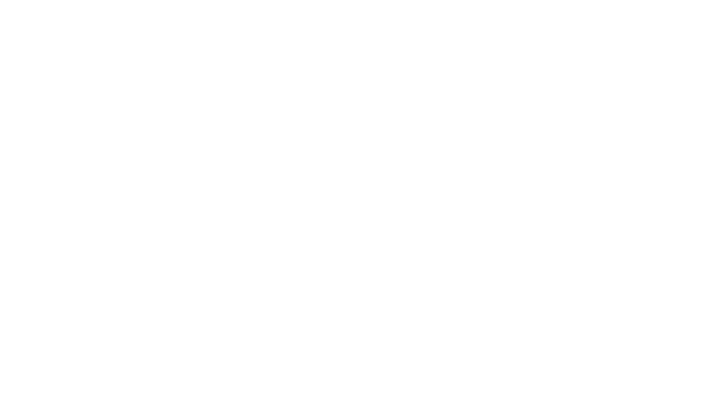Here we see quite a lot of different things:

• Automatically get a beautifully formatted time series along the x axis
• Adding a secondary y axis because our variables have different ranges
• Display article headings on mouseover

``````tds_monthly_totals.iplot(
mode="lines+markers+text",
text=text,
y='word_count',
opacity=0.8,
xTitle="Date",
yTitle="Word Count",
title="Total Word Count by Month")``````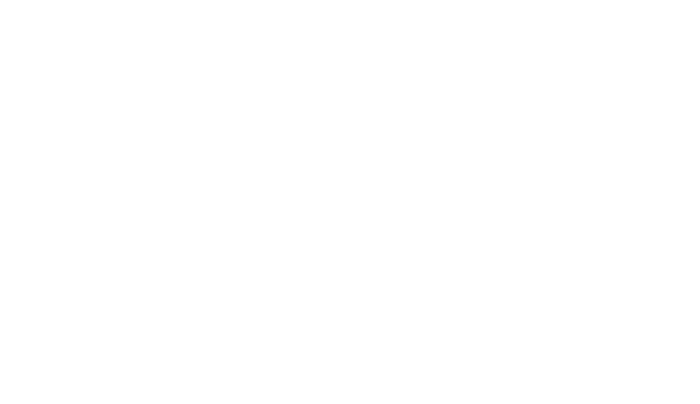For a two-variable scatter chart colored by a third categorical variable, we use:

``````df.iplot(
# Specify the category
categories="publication",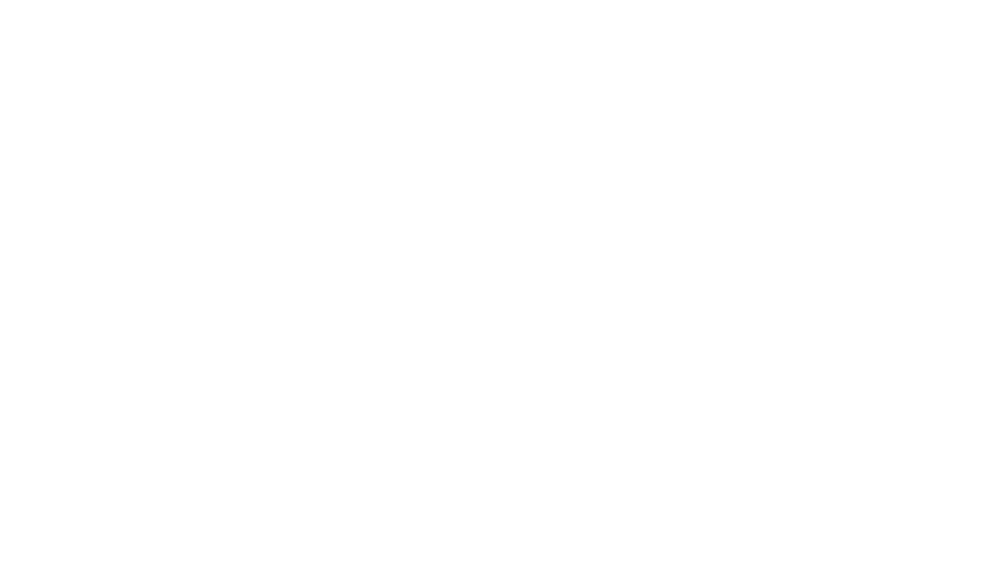Let’s complicate the task a bit using the logarithmic axis defined as a plotly – layout (see Plotly documentation according to the specifications of the layout), and determining the size of the bubbles of a numerical variable:

``````tds.iplot(
x='word_count',
text=text,
mode="markers",
# Log xaxis
layout=dict(
xaxis=dict(type="log", title="Word Count"),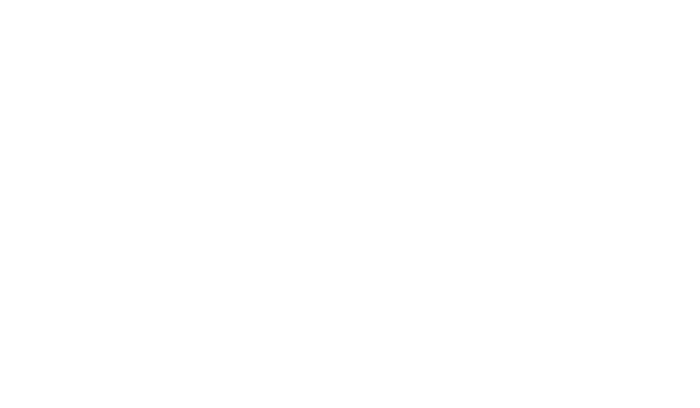Having worked a little (Read more see notebook), we can even put four variables (this is not recommended) on one chart!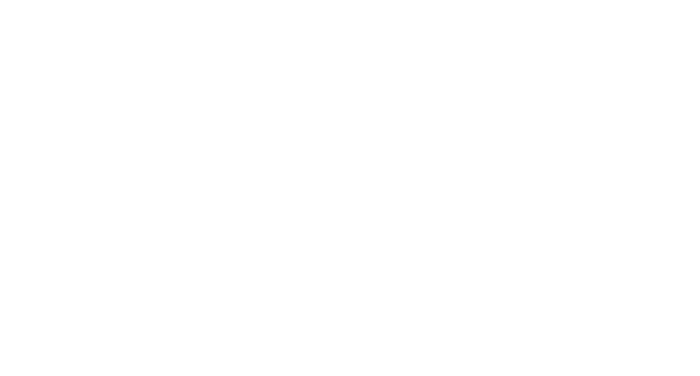As before, we can combine Pandas with plotly + cufflinks for useful graphs.

``````df.pivot_table(
values="views", index='published_date',
columns="publication").cumsum().iplot(
mode="markers+lines",
size=8,
symbol=[1, 2, 3, 4, 5],
layout=dict(
xaxis=dict(title="Date"),
yaxis=dict(type="log", title="Total Views"),
title="Total Views over Time by Publication"))``````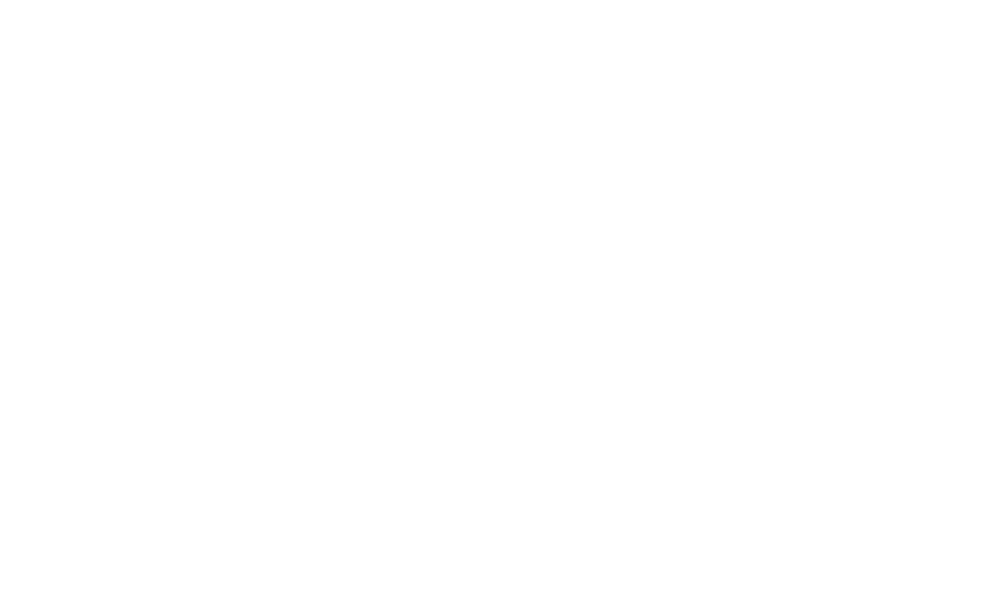For more examples of functionality, see notebook or documentation. We can add text annotations, reference lines and the most suitable lines to our diagrams with a single line of code and still with all interactions.

Now we will move on to a few graphs that you probably won’t use so often, but which can be quite impressive. We will use plotly figure_factoryto make even these incredible gafiks in one line.

### Scattering Matrix

When we want to explore the relationships between many variables, scattering matrix (also called splom) is a great option:

``````import plotly.figure_factory as ff
figure = ff.create_scatterplotmatrix(
df[['claps', 'publication', 'views',
diag='histogram',
index='publication')``````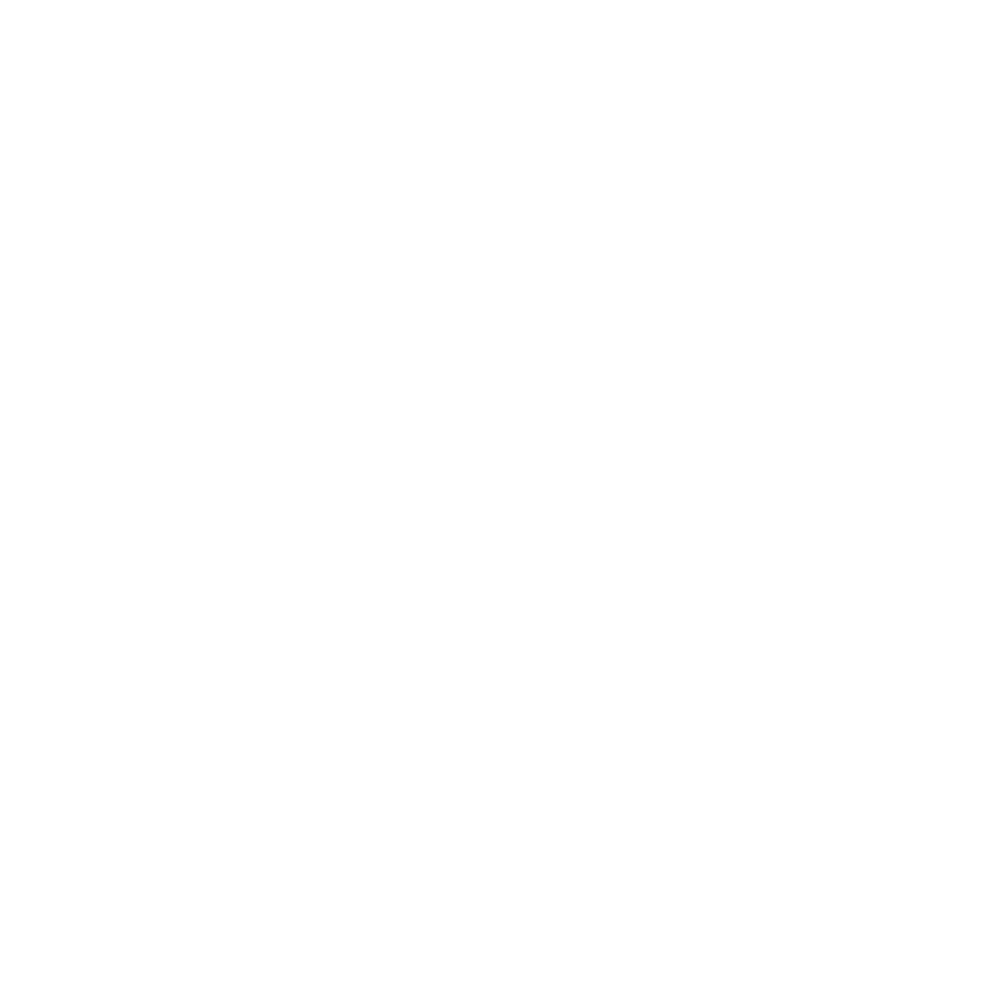Even this graph is fully interactive, which allows us to explore the data.

### Correlation Heat Map

To visualize the correlations between numerical variables, we calculate the correlations, and then make an annotated heat map:

``````corrs = df.corr()
figure = ff.create_annotated_heatmap(
z=corrs.values,
x=list(corrs.columns),
y=list(corrs.index),
annotation_text=corrs.round(2).values,
showscale=True)
``````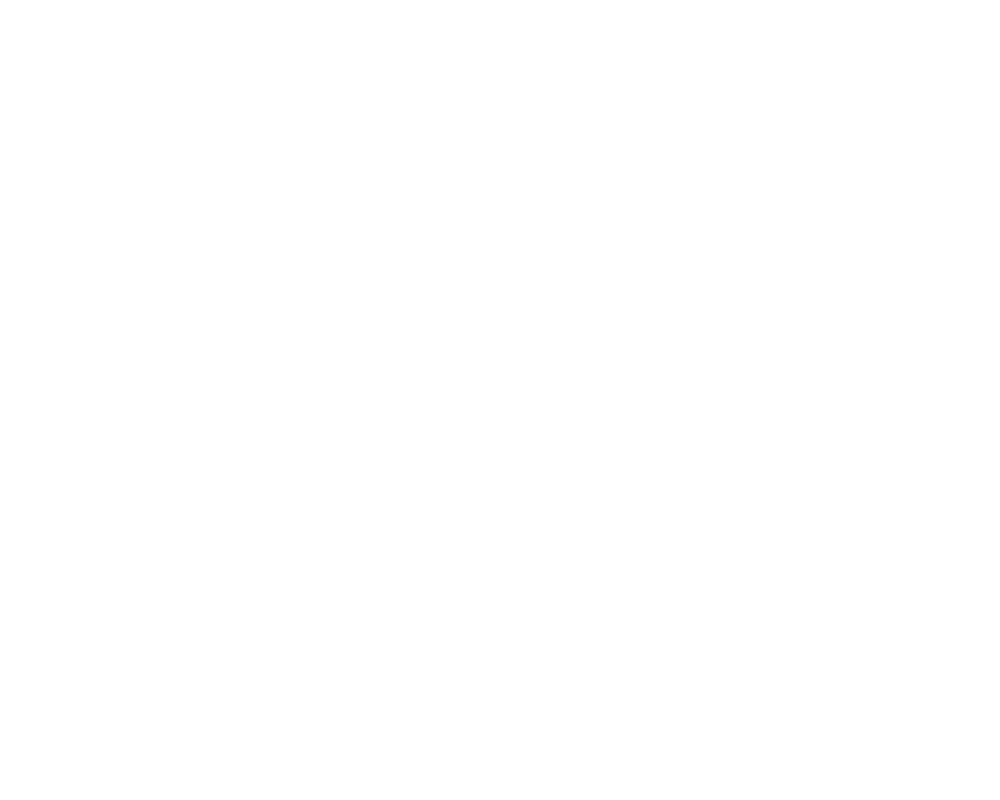The list of charts goes on and on. cufflinks also have several themes that we can use to get a completely different style without any effort. For example, below we have a relationship graph in the “space” topic and a distribution graph in “ggplot”: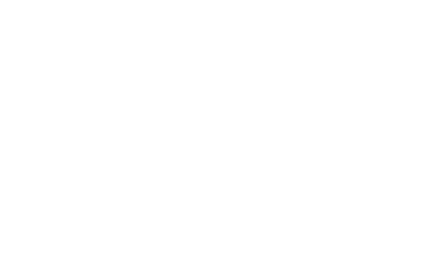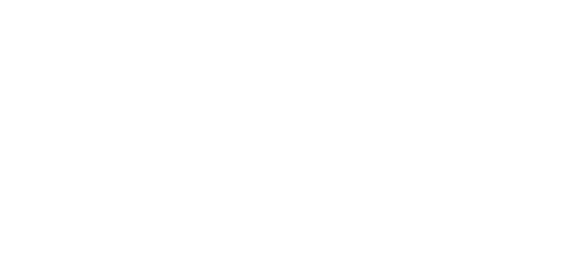We also get 3D graphics (surface and bubble):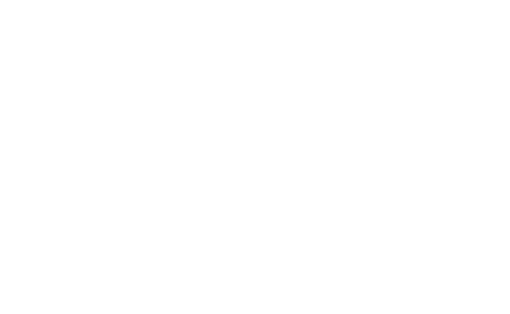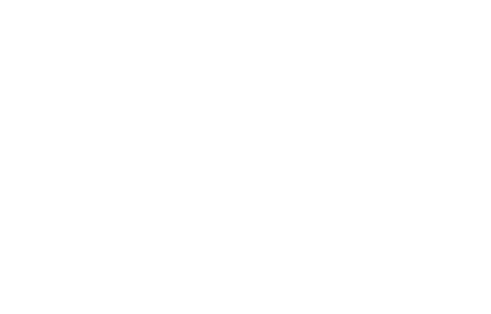For those who want, you can even make a pie chart:### Editing in Plotly Chart Studio

When you draw these graphs in NoteBook Jupiter, you will notice a small link in the lower right corner of the “Export to plot.ly” graph, if you click on this link you will be taken to Chart studio where you can tweak your schedule for the final presentation. You can add annotations, specify colors, and generally clear everything for a great schedule. Then you can publish your schedule on the Internet so that anyone can find it here.

Below are two graphs that I corrected in Chart Studio:Despite everything said here, we still have not explored all the features of the library! I would advise you to look at both the plotly documentation and the cufflinks documentation to build more incredible graphs.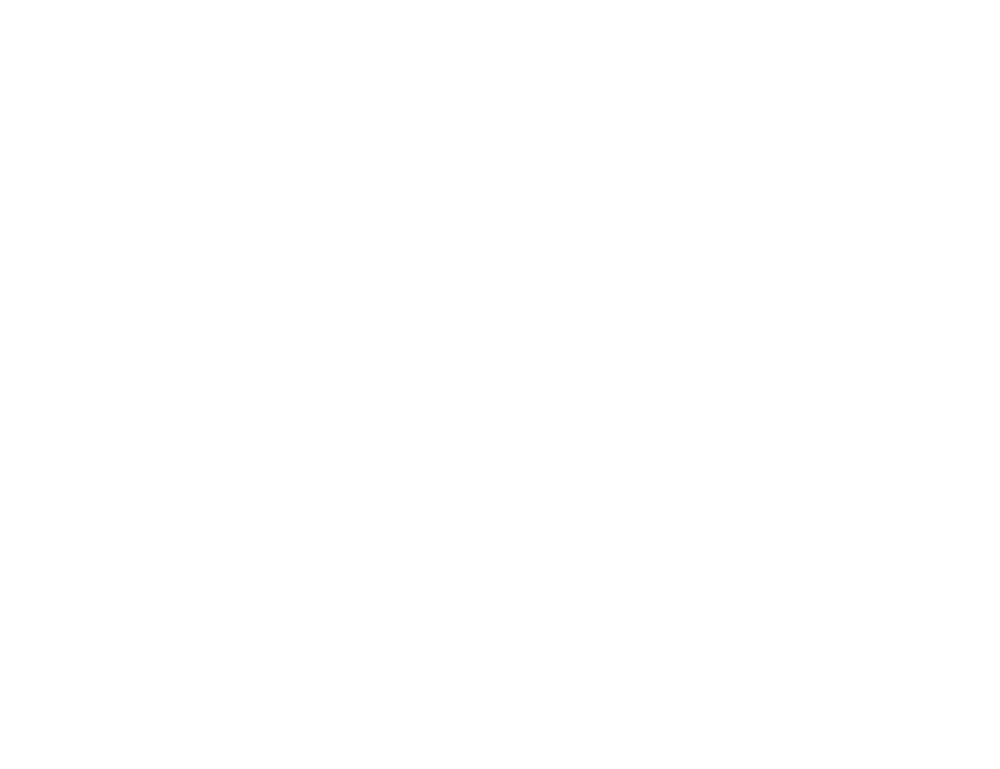### conclusions

The worst part of the undervalued misconception is that you realize how much time you wasted only after you left this business. Fortunately, now that I have made a mistake staying with matploblib for too long, you don’t have to!

When we think about plot libraries, there are a few things we want:

1. Single line graphs for quick exploration
2. Interactive elements for data substitution / exploration
3. The ability to delve into the details as needed
4. Easy setup for the final presentation

For now, the best option for doing all this in Python is plotly. Plotly allows us to quickly render visualizations and helps us better understand our data through interactivity. Also, let’s admit that graphing should be one of the nicest parts of data science! With other libraries, graphing turned into a tedious task, but with plotly again there is joy in creating a great figure!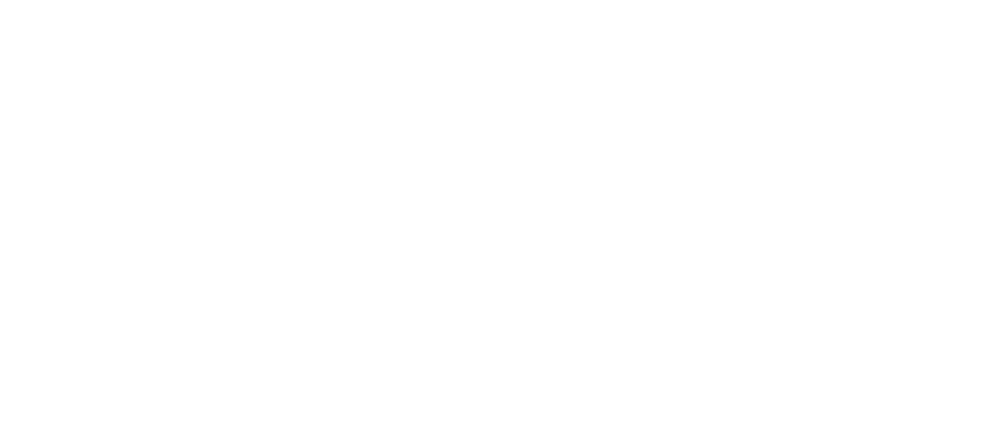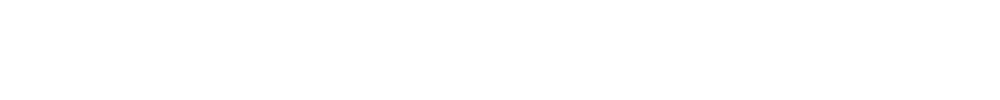Learn the details of how to get a sought-after profession from scratch or Level Up in skills and salary by completing SkillFactory paid online courses: